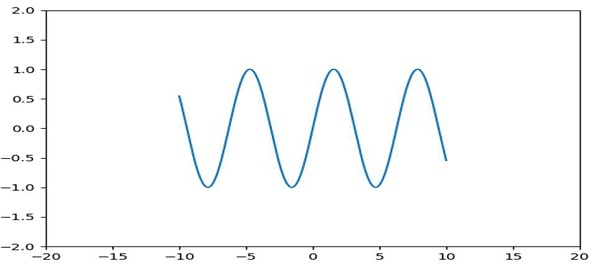# How to enforce axis range in Matplotlib?

To enforce axis range in matplotlib, we can take the following steps −

• Set x and y limits using xlim and ylim methods, respectively.

• Create x and y points for the curve using numpy.

• Plot x and y using the plot() method.

• To show the figure, use the show() method.

## Example

import matplotlib.pyplot as plt
import datetime
import numpy as np
plt.rcParams["figure.figsize"] = [7.50, 3.50]
plt.rcParams["figure.autolayout"] = True
x = np.array([datetime.datetime(2021, 1, 1, i, 0) for i in range(24)])
y = np.random.randint(100, size=x.shape)
plt.plot(x, y)
plt.show()

## Output